# Texas Go Math Grade 3 Lesson 7.4 Answer Key Distributive Property

Refer to our Texas Go Math Grade 3 Answer Key Pdf to score good marks in the exams. Test yourself by practicing the problems from Texas Go Math Grade 3 Lesson 7.4 Answer Key Distributive Property.

## Texas Go Math Grade 3 Lesson 7.4 Answer Key Distributive Property

Essential Question
How can you use the Distributive Property to find products?

Unlock the Problem
Mark bought 6 new fish for his aquarium. He paid $7 for each fish. How much did he spend in all? Find 6 ×$7

• Describe the groups in this problem.
• Circle the numbers you will use to solve the problem.

You can use the Distributive Property to solve the problem.

The Distributive Property states that multiplying a sum by a number is the same as multiplying each addend by the number and then adding the products.

Remember

Activity
Materials,square tiles

Make an array with tiles to show 6 rows of 7.So, Mark spent $___ for his new fish. Break apart the array to make two smaller arrays for facts you know.Math talk Mathematical Processes What other ways could you break apart the 6 × 7 array? Answer: Other ways could you break apart the 6 × 7 array: 6 × 7 = (6 × 4) + (6 × 3) 6 × 7 = (6 × 5) + (6 × 2) 6 × 7 = (6 × 1) + (6 × 6) Explanation: Other ways could you break apart the 6 × 7 array 6 × 7 = (6 × 4) + (6 × 3) = 24 + 16 = 42. 6 × 7 = (6 × 5) + (6 × 2) = 30 + 12 = 42. 6 × 7 = (6 × 1) + (6 × 6) = 6 + 36 = 42. Share and Show Question 1. Draw a line to show how you could break apart this 6 × 8 array into two smaller arrays for facts you know.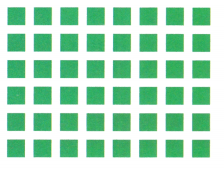• What numbers do you multiply? ___ and ___ ___ and ___ • What numbers do you add? ___ + ___ 6 × 8 = 6 × (___ + ___) 6 × 8 = (___ × ___) + (___ × ____) 6 × 8 = ___ + ____ 6 × 8 = ___ Answer: 6 × 8 = 48.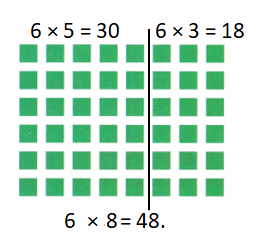Explanation: 6 × 8 = 6 × ( 5+ 3 ) 6 × 8 = ( 6 × 5 ) + ( 6 × 3 ) 6 × 8 = 30 + 18 6 × 8 = 48. Math Talk Mathematical Processes Why do you have to add to find the total product when you use the Distributive Property? Write one way to break apart the array. Then find the product. Answer: 6 × 8 = 48.Explanation: 6 × 8 = 6 × (4 + 4) = (6 × 4) + (6 × 4) = 24 + 24 = 48. Question 2.Answer: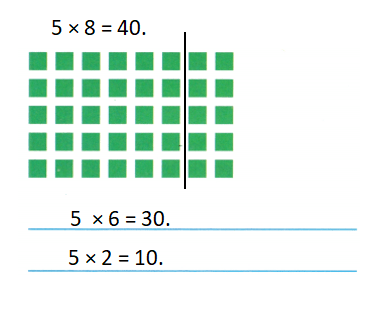Explanation: 5 × 8 = 5 × (6 + 2) = (5 × 6) + (5 × 2) = 30 + 10 = 40. Question 3.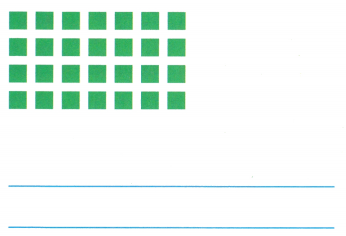Answer: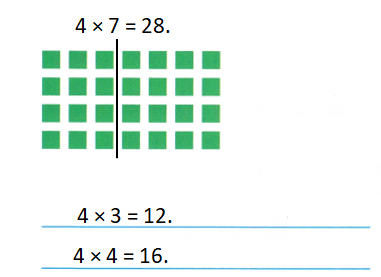Explanation: 4 × 7 = 4 × (3 + 4) = (4 × 3) + (4 × 4) = 12 + 16 = 28. Problem Solving Question 4. Shade tiles to make an array that shows a fact with 7, 8, or 9 as a factor. Write the fact. Explain how you found the product.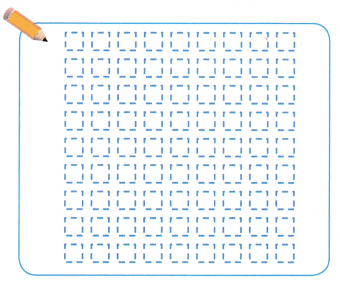Answer:Explanation: Number of rows = 8. Number of columns = 9. Product = Number of rows × Number of columns = 8 × 9 = 72. Problem Solving Question 5. H.O.T. Sense or Nonsense? Robin says, “I can find 8 × 7 by multiplying 3 × 7 and doubling it.” Does her statement make sense? Justify your answer. Answer: Her statement makes sense because 8 × 7 = (3 × 7) + (3 × 7) + (2 × 7) = 56. Explanation: 8 × 7 = (3 + 3 + 2) × 7 = (3 × 7) + (3 × 7) + (2 × 7) = 21 + 21 + 14 = 42 + 14 = 56. Question 6. H.O.T. Multi-Step Brandon needs 8 boxes of spinners for his fishing club. The cost of each box is$9. How much will Brandon pay?8 × $9 = ___ Answer: Amount of money Brandon needs to pay =$72.

Explanation:
Number of boxes of spinners for his fishing club Brandon needs = 8.
Cost of each box = $9. Amount of money Brandon needs to pay = Number of boxes of spinners for his fishing club Brandon needs × Cost of each box = 8 ×$9
= $72. Look at how Brandon solved the problem. Find and describe his error. 8 × 9 = (4 × 9) + (5 × 9) 8 × 9 = 36 + 45 8 × 9 = 81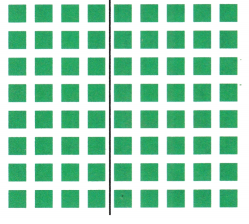Use the array to help solve the problem and correct Brandon’s error. 8 × 9 = (4 + 4) × 9 8 × 9 =(___ × ___) + (__ × ___) 8 × 9 = ___ × ___ 8 × 9 = ____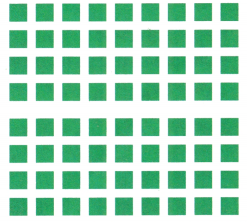So, Brandon will pay$___ for the spinners.
So, Brandon will pay $72 for the spinners. Explanation: 8 × 9 = (4 × 9) + (5 × 9) 8 × 9 = 36 + 45 8 × 9 = 81. Brandon mistake: 8 × 9 = (4 + 4) × 9 not (4 + 5) × 9. Number of boxes of spinners for his fishing club Brandon needs = 8. Cost of each box =$9.
Amount of money Brandon needs to pay = Number of boxes of spinners for his fishing club Brandon needs × Cost of each box
= 8 × $9 = (4 + 4) × 9 = (4 ×$9) + (4 × $9) =$36 + $36 =$72.Fill in the bubble for the correct answer choice.
You may use models or strategies to solve.
Question 7.
Use Diagrams Maurice planted 8 rows of popcorn plants. There are 6 plants in each row. The array shows Maurice’s garden. How many popcorn plants does Maurice have?
(A) 36
(B) 42
(C) 48
(D) 64
Number of popcorn plants Maurice have = 48.
(C) 48.

Explanation:
Number of rows of popcorn plants Maurice planted = 8.
Number of plants in each row = 6.
Number of popcorn plants Maurice have = Number of rows of popcorn plants Maurice planted × Number of plants in each row
= 8 × 6
= (4 + 4) × 6
= (4 × 6) + (4 × 6)
= 24 + 24
= 48.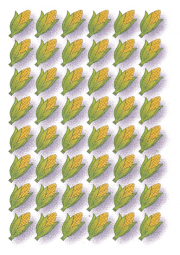Question 8.
At a grocery store, the cans of nuts are arranged in 9 rows. There are 6 cans in each row. Which shows one way to find the total number of cans of nuts?
(A) (5 + 4) × 6
(B) (3 + 3) × 6
(C) (5 + 5) × 6
(D) (5 + 3) × 6
Total number of cans of nuts = 54.
(A) (5 + 4) × 6.

Explanation:
Number of rows of cans of nuts are arranged in = 9.
Number of cans in each row = 6.
Total number of cans of nuts = Number of rows of cans of nuts are arranged in × Number of cans in each row
= 9 × 6
= (5 + 4) × 6
= (5 × 6) + (4 × 6)
= 30 + 24
= 54.

Question 9.
Multi-Step Jillian bought 6 books for $7 each. She paid with$50. How much change should Jillian receive?
(A) $37 (B)$18
(C) $43 (D)$8
Amount of change Jillian receives = $8. (D)$8.

Explanation:
Number of books Jillian bought = 6.
Cost of each book = $7. Amount of money she paid =$50.
Total amount of books Jillian bought = Number of books Jillian bought × Cost of each book
= 6 × $7 = (3 + 3) ×$7
= (3 × $7) + (3 ×$7)
= $21 +$21
= $42. Amount of change Jillian receives = Amount of money she paid – Total amount of books Jillian bought =$50 – $42 =$8.

Texas Lest Prep
Question 10.
Representation Which number sentence below is an example of the Distributive Property?
(A) 6 × 8 = 40 + 8
(B) (8 × 2) × 3 = 8 × (2 × 3)
(C) 6 × 8 = 8 × 6
(D) 6 × 8 = (6 × 2) + (6 × 6)
(B) (8 × 2) × 3 = 8 × (2 × 3) number sentence is an example of the Distributive Property.

Explanation:
In mathematics,  the distributive property of  binary operations generalizes the distributive law, which asserts that the equality.

### Texas Go Math Grade 3 Lesson 7.4 Homework and Practice Answer Key

Write one way to break apart the array. Then find the product.
Question 1.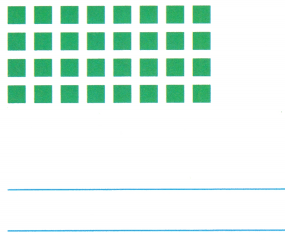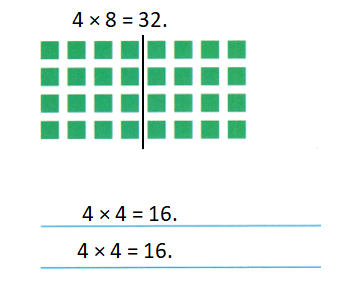Explanation:
4 × 8 = 4 × (4 + 4)
= (4 × 4) + (4 × 4)
= 16 + 16
= 32.

Question 2.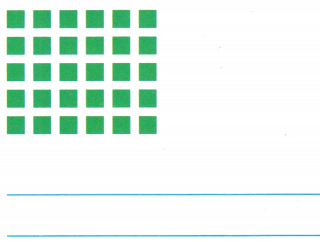Explanation:
5 × 6 = 5 × (2 + 4)
=(5 × 2) + (5 × 4)
= 10 + 20
= 30.

Question 3.
Shade tiles to make an array that shows a fact with 5, 6, or 7 as a factor. Explain how you found the product.Explanation:
Number of rows = 5.
Number of columns = 6.
Product = Number of rows × Number of columns
= 5 × 6
= 5 × (5 + 1)
= (5 × 5) + (5 × 1)
= 25 + 5
= 30.

Problem Solving
Question 4.
Shawn makes a tile design by using 7 rows of 8 tiles. Explain how Shawn can break apart his design to find how many tiles he used.
Number of tiles he used = 7 × 8 = (4 + 3) × 8 = 56.

Explanation:
Number of rows  of tiles design Shawn makes = 7.
Number of tiles in each row = 8.
Number of tiles he used = Number of rows  of tiles design Shawn makes × Number of tiles in each row
= 7 × 8
= (4 + 3) × 8
= (4 × 8) + (3 × 8)
= 32 + 24
= 56.

Texas Test Prep
Lesson Check
Question 5.
There are 8 tables in the art class. Five students sit at each table. How many students are in the art class?
(A) 13
(B) 40
(C) 45
(D) 85
Number of students are in the art class = 40.
(B) 40.

Explanation:
Number of tables in the art class = 8.
Number of students sit at each table = 5.
Number of students are in the art class = Number of tables in the art class × Number of students sit at each table
= 8 × 5
= (5 + 3) × 5
= (5 × 5) + (3 × 5)
= 25 + 15
= 40.

Question 6.
Seven large trucks are in a parking lot. Each truck has 6 wheels. How many wheels are there in all?
(A) 67
(B) 40
(C) 42
(D) 13
Number of wheels are there in all = 42.
(C) 42.

Explanation:
Number of large trucks are in a parking lot = 7.
Number of wheels each truck has = 6.
Number of wheels are there in all = Number of large trucks are in a parking lot × Number of wheels each truck has
= 7 × 6
= (3 + 4) × 6
= (3 × 6) + (4 × 6)
= 18 + 24
= 42.

Question 7.
Jonas buys 6 packages of corn. Each package has 8 ears of corn. Which way can Jonas use to show how many ears of corn he buys?
(A) (4 + 2) × 8
(B) (3 + 2) × 8
(C) (6 + 6) × 8
(D) (4 + 4) × 6
Number of ears of corn he buys = 48.
(A) (4 + 2) × 8

Explanation:
Number of packages of corn Jonas buys = 6.
Number of ears of corns each package has = 8.
Number of ears of corn he buys = Number of packages of corn Jonas buys × Number of ears of corns each package has
= 6 × 8
= (4 + 2) × 8
= (4 × 8) + (2 × 8)
= 32 + 16
= 48.

Question 8.
A store sells bags of 9 apples. Mr. Hyon buys 5 bags of apples to make pies. Which way can Mr. Hyon use to show how many apples he buys?
(A) (4 × 9) + (5 × 9)
(B) (3 × 5) + (6 × 5)
(C) (4 × 5) + (5 × 9)
(D) (3 × 5) + (9 × 5)
Number of apples he buys = 45.
(B) (3 × 5) + (6 × 5)

Explanation:
Number of apples a store sells bags has = 9.
Number of bags of apples to make pies Mr. Hyon buys = 5.
Number of apples he buys = Number of apples a store sells bags has × Number of bags of apples to make pies Mr. Hyon buys
= 9 × 5
= (3 + 6) × 5
= (3 × 5) + (6 × 5)
= 15 + 30
= 45.

Question 9.
Multi-Step Minka buys 8 CDs for $6 each. She pays with three$20 bills. How much change should Minka receive?
(A) $48 (B)$12
(C) $34 (D)$68
Amount of money change Minka receives = $12. (B)$12.

Explanation:
Number of CDs Minka buys = 8.
Cost of each CDs Minka buys = $6. Amount of 3 bills she pays =$20 × 3 = $60. Amount of money change Minka receives = Amount of bills she pays – (Number of CDs Minka buys × Cost of each CDs Minka buys) =$60 – (8 × $6) =$60 – $48 =$12.

Question 10.
Multi-Step Kent buys 5 bags of potatoes that cost $7 each. He gives the clerk$40. How much change should Kent receive?
(A) $35 (B)$12
(C) $68 (D)$5
Amount of change Kent receives = $5. (D)$5.
Cost of each bags of potatoes = $7. Amount of money he gives the clerk =$40.
= $40 – (5 ×$7)
= $40 –$35